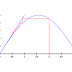This method is also known as direct substitution method or method of iteration or method of fixed iteration.
It is applicable if the equation f(x) = 0 can be expressed as x=g(x).
If x0 id the initial approximation to the root, then the next approximation to the root is given by,
x1=g(x0)
and the next iteration will be
x2= g(x1)

In general,
xn+1=g(xn)

This method converges if |g(x0)|<1 p="">

#include
#include
#include
float g(float x){
return (sqrt(2*x+8));
}
void main(){
int i,n;
float x0,x1;
clrscr();
printf("Enter the no of interation:");
scanf("%d",&n);
printf("Enter the initial value:");
scanf("%f",&x0);
for (i=0;ix1=g(x0);
x0=x1;
}
printf("the reqd. root is:%f",x1);
getch();
}

Fixed Point Iteration MethodReviewed by Santosh Adhikari on October 07, 2018 Rating: 5